# Existence and multiplicity of positive solutions to a perturbed singular elliptic system deriving from a strongly coupled critical potential

## Abstract

In this paper, we consider singular elliptic systems involving a strongly coupled critical potential and concave nonlinearities. By using variational methods and analytical techniques, the existence and multiplicity of positive solutions to the system are established.

MSC: 35J60, 35B33.

## 1 Introduction and main results

In this paper, we consider the following elliptic system:

$\left\{\begin{array}{c}Lu=\frac{{\eta }_{1}{\alpha }_{1}}{{2}^{\ast }}{|u|}^{{\alpha }_{1}-2}{|v|}^{{\beta }_{1}}u+\frac{{\eta }_{2}{\alpha }_{2}}{{2}^{\ast }}{|u|}^{{\alpha }_{2}-2}{|v|}^{{\beta }_{2}}u+{\sigma }_{1}{|u|}^{q-2}u,\hfill \\ Lv=\frac{{\eta }_{1}{\beta }_{1}}{{2}^{\ast }}{|u|}^{{\alpha }_{1}}{|v|}^{{\beta }_{1}-2}v+\frac{{\eta }_{2}{\beta }_{2}}{{2}^{\ast }}{|u|}^{{\alpha }_{2}}{|v|}^{{\beta }_{2}-2}v+{\sigma }_{2}{|v|}^{q-2}v,\hfill \\ \phantom{\rule{1em}{0ex}}u,v\in {H}_{0}^{1}\left(\mathrm{\Omega }\right),\hfill \end{array}$
(1.1)

where $\mathrm{\Omega }\subset {\mathbb{R}}^{N}$ is a smooth bounded domain such that $0\in \mathrm{\Omega }$, $L:=\left(-\mathrm{\Delta }\cdot -\mu \frac{\cdot }{{|x|}^{2}}\right)$, ${2}^{\ast }:=\frac{2N}{N-2}$ is the critical Sobolev exponent, $\overline{\mu }:={\left(\frac{N-2}{2}\right)}^{2}$ is the best Hardy constant and $H:={H}_{0}^{1}\left(\mathrm{\Omega }\right)$ denotes the completion of ${C}_{0}^{\mathrm{\infty }}\left(\mathrm{\Omega }\right)$ with respect to the norm ${\parallel u\parallel }_{0}={\left({\int }_{\mathrm{\Omega }}{|\mathrm{\nabla }u|}^{2}\phantom{\rule{0.2em}{0ex}}dx\right)}^{\frac{1}{2}}$ and ${H}_{\mu }={H}_{\mu }\left(\mathrm{\Omega }\right)$ is defined as the completion of the ${C}_{0}^{\mathrm{\infty }}\left(\mathrm{\Omega }\right)$ with respect to the norm defined by ${\parallel u\parallel }_{\mu }={\left({\int }_{\mathrm{\Omega }}\left({|\mathrm{\nabla }u|}^{2}-\mu \frac{{u}^{2}}{{|x|}^{2}}\right)\phantom{\rule{0.2em}{0ex}}dx\right)}^{\frac{1}{2}}$ for $\mu <\overline{\mu }$.

Definitions of strongly and weakly coupled terms are as follows.

The terms ${|u|}^{\alpha }$ and ${|v|}^{\beta }$ ($\alpha ,\beta >0$) are weakly coupled, ${|Lu|}^{\alpha }{|Kv|}^{\beta }$ ($\alpha ,\beta >0$) is strongly coupled when L or K is a derivative operator. Thus, ${\eta }_{1}{|u|}^{{\alpha }_{1}}{|v|}^{{\beta }_{1}}+{\eta }_{2}{|u|}^{{\alpha }_{2}}{|v|}^{{\beta }_{2}}$ is strongly coupled when ${\eta }_{1}$ and ${\eta }_{2}$ are positive.

The parameters in (1.1) satisfy the following assumption.

() $N\ge 3$, $0\le \mu <\overline{\mu }$, $1, ${\eta }_{1}+{\eta }_{2}>0$, $0\le {\eta }_{i}<+\mathrm{\infty }$, ${\sigma }_{i}>0$, ${\alpha }_{i},{\beta }_{i}>1$, ${\alpha }_{i}+{\beta }_{i}={2}^{\ast }$, $i=1,2$.

The corresponding energy functional of (1.1) is defined in $H×H$ by

$J\left(u,v\right):=\frac{1}{2}{\int }_{\mathrm{\Omega }}\left({|\mathrm{\nabla }u|}^{2}+{|\mathrm{\nabla }v|}^{2}-\mu \frac{{|u|}^{2}+{|v|}^{2}}{{|x|}^{2}}\right)\phantom{\rule{0.2em}{0ex}}dx-\frac{1}{{2}^{\ast }}Q\left(u,v\right)-\frac{1}{q}K\left(u,v\right),$

where $Q\left(u,v\right):={\int }_{\mathrm{\Omega }}\left({\eta }_{1}{|u|}^{{\alpha }_{1}}{|v|}^{{\beta }_{1}}+{\eta }_{2}{|u|}^{{\alpha }_{2}}{|v|}^{{\beta }_{2}}\right)\phantom{\rule{0.2em}{0ex}}dx$ and $K\left(u,v\right):={\int }_{\mathrm{\Omega }}\left({\sigma }_{1}{|u|}^{q}+{\sigma }_{2}{|v|}^{q}\right)\phantom{\rule{0.2em}{0ex}}dx$. Then $J\in {C}^{1}\left(H×H,\mathbb{R}\right)$ and the duality product between $H×H$ and its dual space ${\left(H×H\right)}^{-1}$ is defined as

$\begin{array}{rcl}〈{J}^{\prime }\left(u,v\right),\left({\varphi }_{1},{\varphi }_{2}\right)〉& :=& {\int }_{\mathrm{\Omega }}\left(\mathrm{\nabla }u\mathrm{\nabla }{\varphi }_{1}+\mathrm{\nabla }v\mathrm{\nabla }{\varphi }_{2}-\mu \frac{u{\varphi }_{1}+v{\varphi }_{2}}{{|x|}^{2}}\right)\phantom{\rule{0.2em}{0ex}}dx\\ -{\int }_{\mathrm{\Omega }}\left(\frac{{\eta }_{1}{\alpha }_{1}}{{2}^{\ast }}{|u|}^{{\alpha }_{1}-2}{|v|}^{{\beta }_{1}}u{\varphi }_{1}+\frac{{\eta }_{2}{\alpha }_{2}}{{2}^{\ast }}{|u|}^{{\alpha }_{2}-2}{|v|}^{{\beta }_{2}}u{\varphi }_{1}\right)\phantom{\rule{0.2em}{0ex}}dx\\ -{\int }_{\mathrm{\Omega }}\left(\frac{{\eta }_{1}{\beta }_{1}}{{2}^{\ast }}{|u|}^{{\alpha }_{1}}{|v|}^{{\beta }_{1}-2}v{\varphi }_{2}+\frac{{\eta }_{2}{\beta }_{2}}{{2}^{\ast }}{|u|}^{{\alpha }_{2}}{|v|}^{{\beta }_{2}-2}v{\varphi }_{2}\right)\phantom{\rule{0.2em}{0ex}}dx\\ -{\int }_{\mathrm{\Omega }}\left({\sigma }_{1}{|u|}^{q-2}u{\varphi }_{1}+{\sigma }_{2}{|v|}^{q-2}v{\varphi }_{2}\right)\phantom{\rule{0.2em}{0ex}}dx,\end{array}$

where $u,v,{\varphi }_{1},{\varphi }_{2}\in H$ and ${J}^{\prime }\left(u,v\right)$ denotes the Fréchet derivative of J at $\left(u,v\right)$. A pair of functions $\left(u,v\right)\in H×H$ is said to be a weak solution of (1.1) if

$\left(u,v\right)\ne \left(0,0\right),\phantom{\rule{2em}{0ex}}〈{J}^{\prime }\left(u,v\right),\left({\varphi }_{1},{\varphi }_{2}\right)〉=0,\phantom{\rule{1em}{0ex}}\mathrm{\forall }\left({\varphi }_{1},{\varphi }_{2}\right)\in H×H.$

Therefore, a weak solution of (1.1) is equivalent to a nonzero critical point of $J\left(u,v\right)$ .

Problem (1.1) is related to the well-known Hardy inequality 

${\int }_{{\mathbb{R}}^{N}}\frac{{|u|}^{2}}{{|x|}^{2}}\phantom{\rule{0.2em}{0ex}}dx\le \frac{1}{\overline{\mu }}{\int }_{{\mathbb{R}}^{N}}{|\mathrm{\nabla }u|}^{2}\phantom{\rule{0.2em}{0ex}}dx,\phantom{\rule{1em}{0ex}}\mathrm{\forall }u\in {C}_{0}^{\mathrm{\infty }}\left({\mathbb{R}}^{N}\right).$
(1.2)

If $\mu <\overline{\mu }$, by (1.2), ${\int }_{\mathrm{\Omega }}\left({|\mathrm{\nabla }u|}^{2}-\mu \frac{{u}^{2}}{{|x|}^{2}}\right)\phantom{\rule{0.2em}{0ex}}dx$ is an equivalent norm of H, the operator L is positive and the first eigenvalue ${\mathrm{\Lambda }}_{1}\left(\mu \right)$ of L and the following best constant are well defined:

$S\left(\mu \right):=\underset{u\in {D}^{1,2}\left({\mathbb{R}}^{N}\right)\setminus \left\{0\right\}}{inf}\frac{{\int }_{{\mathbb{R}}^{N}}\left({|\mathrm{\nabla }u|}^{2}-\mu \frac{{u}^{2}}{{|x|}^{2}}\right)\phantom{\rule{0.2em}{0ex}}dx}{{\left({\int }_{{\mathbb{R}}^{N}}{|u|}^{{2}^{\ast }}\phantom{\rule{0.2em}{0ex}}dx\right)}^{\frac{2}{{2}^{\ast }}}},\phantom{\rule{1em}{0ex}}\mathrm{\forall }\mu \in \left(-\mathrm{\infty },\overline{\mu }\right),$
(1.3)

where ${D}^{1,2}\left({\mathbb{R}}^{N}\right)$ is the completion of ${C}_{0}^{\mathrm{\infty }}\left({\mathbb{R}}^{N}\right)$ with respect to ${\left({\int }_{{\mathbb{R}}^{N}}{|\mathrm{\nabla }u|}^{2}\phantom{\rule{0.2em}{0ex}}dx\right)}^{\frac{1}{2}}$. Note that $S\left(0\right)$ is the well-known best Sobolev constant. For $0\le \mu <\overline{\mu }$, the constant $S\left(\mu \right)$ is achieved by the following extremal functions :

${V}_{\mu ,\epsilon }\left(x\right):={\epsilon }^{\frac{2-N}{2}}{U}_{\mu }\left({\epsilon }^{-1}x\right),\phantom{\rule{1em}{0ex}}\mathrm{\forall }\epsilon >0,$
(1.4)

where ${U}_{\mu }\left(x\right)={U}_{\mu }\left(|x|\right)$ is a radially symmetric function

${U}_{\mu }\left(x\right)={\left(\frac{2N\left(\overline{\mu }-\mu \right)}{\sqrt{\overline{\mu }}}\right)}^{\frac{\sqrt{\overline{\mu }}}{2}}{\left({|x|}^{\frac{\sqrt{\overline{\mu }}-\sqrt{\overline{\mu }-\mu }}{\sqrt{\overline{\mu }}}}+{|x|}^{\frac{\sqrt{\overline{\mu }}+\sqrt{\overline{\mu }-\mu }}{\sqrt{\overline{\mu }}}}\right)}^{-\sqrt{\overline{\mu }}}.$

On the other hand, for any $\mu <\overline{\mu }$, ${\eta }_{1}+{\eta }_{2}>0$, $0\le {\eta }_{i}<\mathrm{\infty }$, ${\alpha }_{i},{\beta }_{i}>1$ and ${\alpha }_{i}+{\beta }_{i}={2}^{\ast }$, $i=1,2$, by the Young and Sobolev inequalities, the following best constants are well defined on the space $\mathfrak{D}={\left({D}^{1,2}\left({\mathbb{R}}^{N}\right)\setminus \left\{0\right\}\right)}^{2}$:

${S}_{\eta ,\beta }\left(\mu \right):=\underset{\left(u,v\right)\in \mathfrak{D}}{inf}\frac{{\int }_{{\mathbb{R}}^{N}}\left({|\mathrm{\nabla }u|}^{2}+{|\mathrm{\nabla }v|}^{2}-\mu \frac{{|u|}^{2}+{|v|}^{2}}{{|x|}^{2}}\right)\phantom{\rule{0.2em}{0ex}}dx}{{\left({\int }_{{\mathbb{R}}^{N}}\left({\eta }_{1}{|u|}^{{\alpha }_{1}}{|v|}^{{\beta }_{1}}+{\eta }_{2}{|u|}^{{\alpha }_{2}}{|v|}^{{\beta }_{2}}\right)\phantom{\rule{0.2em}{0ex}}dx\right)}^{\frac{2}{{2}^{\ast }}}}.$
(1.5)

We define

$f\left(\tau \right):=\frac{1+{\tau }^{2}}{{\left({\eta }_{1}{\tau }^{{\beta }_{1}}+{\eta }_{2}{\tau }^{{\beta }_{2}}\right)}^{\frac{2}{{2}^{\ast }}}},\phantom{\rule{1em}{0ex}}\tau >0.$
(1.6)

Since f is a continuous function on $\left(0,\mathrm{\infty }\right)$ such that ${lim}_{\tau \to {0}^{+}}f\left(\tau \right)={lim}_{\tau \to +\mathrm{\infty }}f\left(\tau \right)=+\mathrm{\infty }$. Then there exists ${\tau }_{0}>0$ such that

$f\left({\tau }_{0}\right):=\underset{\tau >0}{min}f\left(\tau \right)>0.$
(1.7)

Set ${\alpha }_{1}={\beta }_{1}$, ${\alpha }_{2}={\beta }_{2}$, ${\sigma }_{1}={\sigma }_{2}$ and $u=v$. Then (1.1) reduces to the semilinear scalar problems that have been extensively investigated by many authors. See  and the references therein.

Regular semilinear elliptic systems have been studied extensively and many conclusions have been established. For example, Alves et al. studied in  an elliptic system and some important conclusions had been obtained. However, the elliptic systems involving the Hardy inequality have seldom been studied and we only find some results in . Thus it is necessary for us to investigate the related singular systems deeply. Among the references above, the elliptic systems involving the Hardy inequality and concave-convex nonlinearities had been studied only in . In this paper, only the case $1 of (1.1) involving multiple strongly-coupled critical terms is considered.

Let $|\mathrm{\Omega }|$ be the Lebesgue measure of Ω. We define the following constant:

${\mathrm{\Upsilon }}_{1}:={\left(\frac{{2}^{\ast }-q}{{2}^{\ast }-2}{\mathrm{\Lambda }}_{1}^{-\frac{q}{2}}{|\mathrm{\Omega }|}^{1-\frac{q}{2}}\right)}^{-1}{\left(\frac{{2}^{\ast }-q}{2-q}{\left({S}_{\eta ,\beta }\left(\mu \right)\right)}^{-\frac{{2}^{\ast }}{2}}\right)}^{-\frac{2-q}{{2}^{\ast }-2}}.$
(1.8)

Then the main results of this paper can be concluded in the following theorems and the conclusions are new to the best of our knowledge. It can be verified that the intervals in Theorems 1.1 and 1.2 for the parameters ${\sigma }_{1}$, ${\sigma }_{2}$, μ and q are allowable.

Theorem 1.1 Suppose that () holds and ${\sigma }_{1}+{\sigma }_{2}<{\mathrm{\Upsilon }}_{1}$. Then problem (1.1) has at least one positive solution.

Theorem 1.2 Suppose that () holds, $N>4$, $\mu <\overline{\mu }-{\left(\frac{N}{q}-\sqrt{\overline{\mu }}\right)}^{2}$ and $\frac{N}{N-2}. Then there exists ${\mathrm{\Upsilon }}_{2}>0$ such that problem (1.1) has at least two positive solutions for all ${\sigma }_{1}$ and ${\sigma }_{2}$ satisfying ${\sigma }_{1}+{\sigma }_{2}<{\mathrm{\Upsilon }}_{2}$.

This paper is organized as follows. Some preliminary results and properties of the Nehari manifold are established in Sections 2 and 3, and Theorems 1.1 and 1.2 are proved in Section 4.

## 2 The local Palais-Smale condition

Throughout this paper, we always assume that the assumption () holds, ${\parallel u\parallel }_{H}:={\parallel u\parallel }_{\mu }={\left({\int }_{\mathrm{\Omega }}\left({|\mathrm{\nabla }u|}^{2}-\mu \frac{{|u|}^{2}}{{|x|}^{2}}\right)\phantom{\rule{0.2em}{0ex}}dx\right)}^{1/2}$ denotes the norm of the space H, by the Hardy inequality ${\parallel \cdot \parallel }_{\mu }$ is equivalent to ${\parallel \cdot \parallel }_{0}$, i.e.,

${\left(1-\frac{1}{\overline{\mu }}max\left(\mu ,0\right)\right)}^{1/2}{\parallel u\parallel }_{0}\le {\parallel u\parallel }_{\mu }\le {\left(1-\frac{1}{\overline{\mu }}min\left(\mu ,0\right)\right)}^{1/2}{\parallel u\parallel }_{0},\phantom{\rule{1em}{0ex}}\mathrm{\forall }u\in H.$

${\mathrm{\Lambda }}_{1}$ denotes the first eigenvalue of the operator L, $\parallel z\parallel ={\parallel z\parallel }_{H×H}={\parallel \left(u,v\right)\parallel }_{H×H}={\left({\parallel u\parallel }_{H}^{2}+{\parallel v\parallel }_{H}^{2}\right)}^{1/2}$ means the norm of the space $E:={H}_{0}^{1}\left(\mathrm{\Omega }\right)×{H}_{0}^{1}\left(\mathrm{\Omega }\right)$, ${E}^{-1}$ is the dual space of E. $tz=t\left(u,v\right)=\left(tu,tv\right)$ for all $z\in E$ and $t\in \mathbb{R}$. $z=\left(u,v\right)$ is said to be nonnegative in Ω if $u\ge 0$ and $v\ge 0$ in Ω. $z=\left(u,v\right)$ is said to be positive in Ω if $u>0$ and $v>0$ in Ω. ${B}_{r}\left(0\right)=\left\{x\in {\mathbb{R}}^{N}\mid |x| is a ball in ${\mathbb{R}}^{N}$. $O\left({\epsilon }^{t}\right)$ denotes a quantity satisfying $|O\left({\epsilon }^{t}\right)|/{\epsilon }^{t}\le C$, $o\left({\epsilon }^{t}\right)$ means $|o\left({\epsilon }^{t}\right)|/{\epsilon }^{t}\to 0$ as $\epsilon \to 0$ and $o\left(1\right)$ is a generic infinitesimal value. In particular, the quantity ${O}_{1}\left({\epsilon }^{t}\right)$ means that there exist the constants ${C}_{1},{C}_{2}>0$ such that ${C}_{1}{\epsilon }^{t}\le {O}_{1}\left({\epsilon }^{t}\right)\le {C}_{2}{\epsilon }^{t}$ as ε is small. We always denote positive constants as C and omit dx in integrals for convenience.

Lemma 2.1 If $\left\{{z}_{n}\right\}\subset E$ is a (PS) c -sequence of J with ${z}_{n}⇀z$ in E, then ${J}^{\prime }\left(z\right)=0$ and $J\left(z\right)\ge F\left({\tau }_{0}^{\left(1\right)},{\tau }_{0}^{\left(2\right)}\right)$, where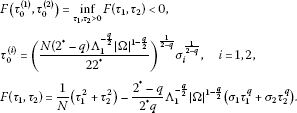Proof Let ${z}_{n}=\left({u}_{n},{v}_{n}\right)$ and $z=\left(u,v\right)$. Since $\left\{{z}_{n}\right\}$ is a (PS) c -sequence of J with ${z}_{n}⇀z$ in E, we can deduce that ${J}^{\prime }\left(z\right)=0$, and therefore $〈{J}^{\prime }\left(z\right),z〉=0$, that is,

$Q\left(z\right)={\parallel z\parallel }^{2}-K\left(z\right).$

Consequently,

$J\left(z\right)=\left(\frac{1}{2}-\frac{1}{{2}^{\ast }}\right){\parallel z\parallel }^{2}-\left(\frac{1}{q}-\frac{1}{{2}^{\ast }}\right)K\left(z\right).$

From the Hölder inequality it follows that

$\begin{array}{rcl}J\left(z\right)& \ge & \left(\frac{1}{2}-\frac{1}{{2}^{\ast }}\right){\parallel z\parallel }^{2}-\left(\frac{1}{q}-\frac{1}{{2}^{\ast }}\right){|\mathrm{\Omega }|}^{1-\frac{q}{2}}\left({\sigma }_{1}{\left({\int }_{\mathrm{\Omega }}{|u|}^{2}\right)}^{\frac{q}{2}}+{\sigma }_{2}{\left({\int }_{\mathrm{\Omega }}{|u|}^{2}\right)}^{\frac{q}{2}}\right)\\ \ge & \frac{1}{N}{\parallel z\parallel }^{2}-\frac{{2}^{\ast }-q}{{2}^{\ast }q}{\mathrm{\Lambda }}_{1}^{-\frac{q}{2}}{|\mathrm{\Omega }|}^{1-\frac{q}{2}}\left({\sigma }_{1}{\parallel u\parallel }_{H}^{q}+{\sigma }_{2}{\parallel v\parallel }_{H}^{q}\right)\\ \ge & F\left({\tau }_{0}^{\left(1\right)},{\tau }_{0}^{\left(2\right)}\right).\end{array}$

Thus, the proof is complete. □

Lemma 2.2 If $\left\{{z}_{n}\right\}\subset E$ is a (PS) c -sequence of the functional J, then $\left\{{z}_{n}\right\}$ is bounded in E.

Proof See Hsu [, Lemma 2.2]. □

Lemma 2.3 Suppose that () holds. Then J satisfies the (PS) c condition for all $c<{c}^{\ast }$, where

${c}^{\ast }=\frac{1}{N}{\left({S}_{\eta ,\beta }\left(\mu \right)\right)}^{\frac{N}{2}}+F\left({\tau }_{0}^{\left(1\right)},{\tau }_{0}^{\left(2\right)}\right).$
(2.1)

Proof We follow the argument in . Let $\left\{{z}_{n}\right\}\subset E$ be a (PS) c -sequence of J with $c<{c}^{\ast }$. Write ${z}_{n}=\left({u}_{n},{v}_{n}\right)$. We know from Lemma 2.2 that $\left\{{z}_{n}\right\}$ is bounded in E, and then ${z}_{n}⇀z=\left(u,v\right)$ up to a subsequence, z is a critical point of J. Furthermore, we may assume that ${u}_{n}⇀u$, ${v}_{n}⇀v$ weakly in H and ${u}_{n}\to u$, ${v}_{n}\to v$ strongly in ${L}^{s}\left(\mathrm{\Omega }\right)$ for all $1\le s<{2}^{\ast }$ and ${u}_{n}\to u$, ${v}_{n}\to v$ a.e. in Ω. Hence, we have that

${J}^{\prime }\left(z\right)=0\phantom{\rule{1em}{0ex}}\text{and}\phantom{\rule{1em}{0ex}}K\left({z}_{n}\right)=K\left(z\right)+o\left(1\right).$
(2.2)

Set ${\stackrel{˜}{u}}_{n}={u}_{n}-u$, ${\stackrel{˜}{v}}_{n}={v}_{n}-v$ and ${\stackrel{˜}{z}}_{n}=\left({\stackrel{˜}{u}}_{n},{\stackrel{˜}{v}}_{n}\right)$. From the Brézis-Lieb lemma  it follows that

${\parallel {\stackrel{˜}{z}}_{n}\parallel }^{2}={\parallel {z}_{n}\parallel }^{2}-{\parallel z\parallel }^{2}+o\left(1\right),$
(2.3)

and by Lemma 2.1 in  we have

${\int }_{\mathrm{\Omega }}{|{\stackrel{˜}{u}}_{n}|}^{{\alpha }_{i}}{|{\stackrel{˜}{v}}_{n}|}^{{\beta }_{i}}={\int }_{\mathrm{\Omega }}{|{u}_{n}|}^{{\alpha }_{i}}{|{v}_{n}|}^{{\beta }_{i}}-{\int }_{\mathrm{\Omega }}{|u|}^{{\alpha }_{i}}{|v|}^{{\beta }_{i}}+o\left(1\right),\phantom{\rule{1em}{0ex}}i=1,2.$
(2.4)

Since $J\left({z}_{n}\right)=c+o\left(1\right)$, ${J}^{\prime }\left({z}_{n}\right)=o\left(1\right)$ and by (2.2) to (2.4), we can deduce that

$\frac{1}{2}{\parallel {\stackrel{˜}{z}}_{n}\parallel }^{2}-\frac{1}{{2}^{\ast }}Q\left({\stackrel{˜}{z}}_{n}\right)=c-J\left(z\right)+o\left(1\right)$
(2.5)

and

${\parallel {\stackrel{˜}{z}}_{n}\parallel }^{2}-Q\left({\stackrel{˜}{z}}_{n}\right)=o\left(1\right).$

Hence, we may assume that

$\underset{n\to \mathrm{\infty }}{lim}{\parallel {\stackrel{˜}{z}}_{n}\parallel }^{2}=\underset{n\to \mathrm{\infty }}{lim}Q\left({\stackrel{˜}{z}}_{n}\right)=l.$
(2.6)

If $l=0$, the proof is complete. Assume $l>0$; then from (2.6) and the definition of ${S}_{\eta ,\beta }\left(\mu \right)$ it follows that

$\begin{array}{rcl}{S}_{\eta ,\beta }\left(\mu \right){l}^{\frac{2}{{2}^{\ast }}}& =& \underset{n\to \mathrm{\infty }}{lim}{S}_{\eta ,\beta }\left(\mu \right){\left(Q\left({\stackrel{˜}{z}}_{n}\right)\right)}^{\frac{2}{{2}^{\ast }}}\\ \le & \underset{n\to \mathrm{\infty }}{lim}{\parallel {\stackrel{˜}{z}}_{n}\parallel }^{2}=l,\end{array}$

which implies that

$l\ge {\left({S}_{\eta ,\beta }\left(\mu \right)\right)}^{\frac{N}{2}}.$
(2.7)

In addition, from (2.5) to (2.7) and Lemma 2.1, we get

$\begin{array}{rcl}c& =& \left(\frac{1}{2}-\frac{1}{{2}^{\ast }}\right)l+J\left(z\right)\\ \ge & \frac{1}{N}{\left({S}_{\eta ,\beta }\left(\mu \right)\right)}^{\frac{N}{2}}+F\left({\tau }_{0}^{\left(1\right)},{\tau }_{0}^{\left(2\right)}\right)={c}^{\ast },\end{array}$

which is a contradiction. Therefore, the proof of Lemma 2.3 is complete. □

## 3 Nehari manifold

Since J is unbounded below on E, we need to consider J on the Nehari manifold

${\mathcal{M}}_{\sigma }=\left\{z\in E\setminus \left\{0\right\}:〈{J}^{\prime }\left(z\right),z〉=0\right\}.$

Thus, $z\in {\mathcal{M}}_{\sigma }$ if and only if

$〈{J}^{\prime }\left(z\right),z〉={\parallel z\parallel }^{2}-Q\left(z\right)-K\left(z\right)=0.$
(3.1)

By the Hölder inequality and the definition of ${\mathrm{\Lambda }}_{1}$ it follows that

$\begin{array}{rcl}K\left(z\right)& \le & \left({\sigma }_{1}{\left({\int }_{\mathrm{\Omega }}{|u|}^{2}\right)}^{\frac{q}{2}}+{\sigma }_{2}{\left({\int }_{\mathrm{\Omega }}{|v|}^{2}\right)}^{\frac{q}{2}}\right){|\mathrm{\Omega }|}^{1-\frac{q}{2}}\\ \le & \left({\sigma }_{1}{\left({\parallel u\parallel }_{H}\right)}^{q}+{\sigma }_{2}{\left({\parallel v\parallel }_{H}\right)}^{q}\right){\mathrm{\Lambda }}_{1}^{-\frac{q}{2}}{|\mathrm{\Omega }|}^{1-\frac{q}{2}}\\ \le & \left({\sigma }_{1}+{\sigma }_{2}\right){\mathrm{\Lambda }}_{1}^{-\frac{q}{2}}{|\mathrm{\Omega }|}^{1-\frac{q}{2}}{\parallel z\parallel }^{q}.\end{array}$
(3.2)

Lemma 3.1 The functional J is coercive and bounded below on ${\mathcal{M}}_{\sigma }$.

Proof Suppose that $z=\left(u,v\right)\in {\mathcal{M}}_{\sigma }$. From (3.1) and (3.2) we get

$J\left(z\right)=\left(\frac{1}{2}-\frac{1}{{2}^{\ast }}\right){\parallel z\parallel }^{2}-\left(\frac{1}{q}-\frac{1}{{2}^{\ast }}\right)K\left(z\right)$
(3.3)
$\ge \frac{1}{N}{\parallel z\parallel }^{2}-\left(\frac{{2}^{\ast }-q}{{2}^{\ast }q}\right)\left({\sigma }_{1}+{\sigma }_{2}\right){\mathrm{\Lambda }}_{1}^{-\frac{q}{2}}{|\mathrm{\Omega }|}^{1-\frac{q}{2}}{\parallel z\parallel }^{q}.$
(3.4)

Thus, J is coercive and bounded below on ${\mathcal{M}}_{\sigma }$. □

Define $\mathrm{\Phi }\left(z\right)=〈{J}^{\prime }\left(z\right),z〉$. Then for all $z=\left(u,v\right)\in {\mathcal{M}}_{\sigma }$ we have

$\begin{array}{rcl}〈{\mathrm{\Phi }}^{\prime }\left(z\right),z〉& =& 2{\parallel z\parallel }^{2}-{2}^{\ast }Q\left(z\right)-qK\left(z\right)\\ =& \left(2-q\right){\parallel z\parallel }^{2}-\left({2}^{\ast }-q\right)Q\left(z\right)\end{array}$
(3.5)
$=-\left({2}^{\ast }-2\right){\parallel z\parallel }^{2}+\left({2}^{\ast }-q\right)K\left(z\right).$
(3.6)

We split ${\mathcal{M}}_{\sigma }$ into three parts: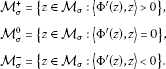Lemma 3.2 Suppose that $z\in E$ is a local minimizer of J on ${\mathcal{M}}_{\sigma }$ and $z\notin {\mathcal{M}}_{\sigma }^{0}$. Then ${J}^{\prime }\left(z\right)=0$ in ${E}^{-1}$.

Proof The proof is similar to that of  and the details are omitted. □

Lemma 3.3 ${\mathcal{M}}_{\sigma }^{0}=\mathrm{\varnothing }$ for all ${\sigma }_{1}+{\sigma }_{2}\in \left(0,{\mathrm{\Upsilon }}_{1}\right)$.

Proof We argue by contradiction. Suppose that there exist ${\sigma }_{1},{\sigma }_{2}>0$ such that $0<{\sigma }_{1}+{\sigma }_{2}<{\mathrm{\Upsilon }}_{1}$ and ${\mathcal{M}}_{\sigma }^{0}\ne \mathrm{\varnothing }$. Then the fact $z=\left(u,v\right)\in {\mathcal{M}}_{\sigma }^{0}$ together with (3.5) and (3.6) imply that

${\parallel z\parallel }^{2}=\frac{{2}^{\ast }-q}{2-q}Q\left(z\right),$
(3.7)

and

${\parallel z\parallel }^{2}=\frac{{2}^{\ast }-q}{{2}^{\ast }-2}K\left(z\right).$
(3.8)

By (1.5) and (3.7) we have

${\parallel z\parallel }^{2}\le \frac{{2}^{\ast }-q}{2-q}{\left({S}_{\eta ,\beta }\left(\mu \right)\right)}^{-\frac{{2}^{\ast }}{2}}{\parallel z\parallel }^{{2}^{\ast }},$

which implies that

$\parallel z\parallel \ge {\left(\frac{{2}^{\ast }-q}{2-q}{\left({S}_{\eta ,\beta }\left(\mu \right)\right)}^{-\frac{{2}^{\ast }}{2}}\right)}^{-\frac{1}{{2}^{\ast }-2}}.$
(3.9)

By (3.2) and (3.8) we have

$\parallel z\parallel \le {\left(\frac{{2}^{\ast }-q}{{2}^{\ast }-2}{\mathrm{\Lambda }}_{1}^{-\frac{q}{2}}{|\mathrm{\Omega }|}^{1-\frac{q}{2}}\right)}^{\frac{1}{2-q}}{\left({\sigma }_{1}+{\sigma }_{2}\right)}^{\frac{1}{2-q}}.$
(3.10)

From (3.9) and (3.10) it follows that

${\sigma }_{1}+{\sigma }_{2}\ge {\left(\frac{{2}^{\ast }-q}{{2}^{\ast }-2}{\mathrm{\Lambda }}_{1}^{-\frac{q}{2}}{|\mathrm{\Omega }|}^{1-\frac{q}{2}}\right)}^{-1}{\left(\frac{{2}^{\ast }-q}{2-q}{\left({S}_{\eta ,\beta }\left(\mu \right)\right)}^{-\frac{{2}^{\ast }}{2}}\right)}^{-\frac{2-q}{{2}^{\ast }-2}}={\mathrm{\Upsilon }}_{1},$

By Lemma 3.3, we write ${\mathcal{M}}_{\sigma }={\mathcal{M}}_{\sigma }^{+}\cup {\mathcal{M}}_{\sigma }^{-}$ and define

${\alpha }_{\sigma }=\underset{z\in {\mathcal{M}}_{\sigma }}{inf}J\left(z\right);\phantom{\rule{2em}{0ex}}{\alpha }_{\sigma }^{±}=\underset{z\in {\mathcal{M}}_{\sigma }^{±}}{inf}J\left(z\right).$

Lemma 3.4

1. (i)

${\alpha }_{\sigma }\le {\alpha }_{\sigma }^{+}<0$ for all ${\sigma }_{1}+{\sigma }_{2}\in \left(0,{\mathrm{\Upsilon }}_{1}\right)$.

2. (ii)

There exists a positive constant ${d}_{0}$ depending on ${\sigma }_{1}$, ${\sigma }_{2}$, q, N, ${S}_{\eta ,\beta }\left(\mu \right)$, ${\mathrm{\Lambda }}_{1}$ and $|\mathrm{\Omega }|$ such that ${\alpha }_{\sigma }^{-}>{d}_{0}$ for all ${\sigma }_{1}+{\sigma }_{2}\in \left(0,\frac{q}{2}{\mathrm{\Upsilon }}_{1}\right)$.

Proof (i) Let $z=\left(u,v\right)\in {\mathcal{M}}_{\sigma }^{+}$. By (3.1) and (3.6) it follows that

$\frac{2-q}{{2}^{\ast }-q}{\parallel z\parallel }^{2}>Q\left(z\right).$
(3.11)

According to (3.1) and (3.11), we have that

$\begin{array}{rl}J\left(z\right)& =\left(\frac{1}{2}-\frac{1}{q}\right){\parallel z\parallel }^{2}+\left(\frac{1}{q}-\frac{1}{{2}^{\ast }}\right)Q\left(z\right)\\ <\left[\left(\frac{1}{2}-\frac{1}{q}\right)+\left(\frac{1}{q}-\frac{1}{{2}^{\ast }}\right)\frac{2-q}{{2}^{\ast }-q}\right]{\parallel z\parallel }^{2}\\ =-\frac{2-q}{qN}{\parallel z\parallel }^{2}<0,\end{array}$

which implies that ${\alpha }_{\sigma }\le {\alpha }_{\sigma }^{+}<0$.

1. (ii)

Suppose that ${\sigma }_{1}+{\sigma }_{2}\in \left(0,\frac{q}{2}{\mathrm{\Upsilon }}_{1}\right)$ and $z=\left(u,v\right)\in {\mathcal{M}}_{\sigma }^{-}$. By (1.7), (3.1) and (3.5) we have that

$\frac{2-q}{{2}^{\ast }-q}\parallel z\parallel

which implies that

$\parallel z\parallel >{\left(\frac{2-q}{{2}^{\ast }-q}\right)}^{\frac{1}{{2}^{\ast }-2}}{\left({S}_{\eta ,\beta }\left(\mu \right)\right)}^{\frac{{2}^{\ast }}{2\left({2}^{\ast }-2\right)}}.$
(3.12)

From (3.4) and (3.12) it follows that

$J\left(z\right)\ge {\parallel z\parallel }^{q}\left(\frac{1}{N}{\parallel z\parallel }^{2-q}-\left(\frac{{2}^{\ast }-q}{{2}^{\ast }q}\right){\mathrm{\Lambda }}_{1}^{-\frac{q}{2}}{|\mathrm{\Omega }|}^{1-\frac{q}{2}}\left({\sigma }_{1}+{\sigma }_{2}\right)\right)\ge {d}_{0},$

where ${d}_{0}={d}_{0}\left({\sigma }_{1},{\sigma }_{2},q,N,{\mathrm{\Lambda }}_{1},{S}_{\eta ,\beta }\left(\mu \right),|\mathrm{\Omega }|\right)$ is a positive constant. □

Lemma 3.5 Suppose that ${\sigma }_{1}+{\sigma }_{2}\in \left(0,{\mathrm{\Upsilon }}_{1}\right)$ and $z\in E$ with $Q\left(z\right)>0$. Then there exist unique ${t}^{+},{t}^{-}>0$ such that ${t}^{+}z\in {\mathcal{M}}_{\sigma }^{+}$ and ${t}^{-}z\in {\mathcal{M}}_{\sigma }^{-}$. In particular, we have

${t}^{-}>{t}_{\mathrm{max}}:={\left(\frac{\left(2-q\right){\parallel z\parallel }^{2}}{\left({2}^{\ast }-q\right)Q\left(z\right)}\right)}^{\frac{1}{{2}^{\ast }-2}}>{t}^{+},$

$J\left({t}^{+}z\right)={min}_{0\le t\le {t}_{\mathrm{max}}}J\left(tz\right)$ and $J\left({t}^{-}z\right)={max}_{t\ge {t}_{\mathrm{max}}}J\left(tz\right)$.

Proof The proof is similar to that of  and is omitted. □

For each $z\in E$ with $K\left(z\right)>0$, we write

${\overline{t}}_{\mathrm{max}}={\left(\frac{\left({2}^{\ast }-q\right)K\left(z\right)}{\left({2}^{\ast }-2\right){\parallel z\parallel }^{2}}\right)}^{\frac{1}{2-q}}>0.$

Then we have the following lemma.

Lemma 3.6 Suppose that ${\sigma }_{1}+{\sigma }_{2}\in \left(0,{\mathrm{\Upsilon }}_{1}\right)$ and $z\in E\setminus \left\{\left(0,0\right)\right\}$ with $K\left(z\right)>0$. Then there exist unique $0<{t}^{+}<{\overline{t}}_{\mathrm{max}}<{t}^{-}$ such that ${t}^{+}z\in {\mathcal{M}}_{\sigma }^{+}$, ${t}^{-}z\in {\mathcal{M}}_{\sigma }^{-}$ and

$J\left({t}^{+}z\right)=\underset{0\le t\le {\overline{t}}_{\mathrm{max}}}{inf}J\left(tz\right);J\left({t}^{-}z\right)=\underset{t\ge 0}{sup}J\left(tz\right).$

Proof The proof is almost the same as that in [, Lemma 2.7] and is omitted here. □

## 4 Proof of Theorems 1.1 and 1.2

Lemma 4.1

1. (i)

If ${\sigma }_{1}+{\sigma }_{2}\in \left(0,{\mathrm{\Upsilon }}_{1}\right)$, then the functional J has a (PS)-sequence $\left\{{z}_{n}\right\}\subset {\mathcal{M}}_{\sigma }$.

2. (ii)

If ${\sigma }_{1}+{\sigma }_{2}\in \left(0,\frac{q}{2}{\mathrm{\Upsilon }}_{1}\right)$, then the functional J has a ${\text{(}\mathit{\text{PS}}\text{)}}_{{\alpha }_{\sigma }^{-}}$-sequence $\left\{{z}_{n}\right\}\subset {\mathcal{M}}_{\sigma }^{-}$.

Proof The proof is similar to that of  and is omitted. □

Lemma 4.2 Suppose that ${\sigma }_{1}+{\sigma }_{2}\in \left(0,{\mathrm{\Upsilon }}_{1}\right)$. Then J has a minimizer ${z}^{\left(1\right)}\in {\mathcal{M}}_{\sigma }^{+}$ such that ${z}^{\left(1\right)}$ is a positive solution of (1.1) and $J\left({z}^{\left(1\right)}\right)={\alpha }_{\sigma }={\alpha }_{\sigma }^{+}<0$.

Proof By Lemma 4.1(i), there exists a (PS)-sequence $\left\{{z}_{n}\right\}\subset {\mathcal{M}}_{\sigma }$ of J such that

(4.1)

Since J is coercive on ${\mathcal{M}}_{\sigma }$ (see Lemma 3.1), we get that $\left\{{z}_{n}\right\}$ is bounded in E. Passing to a subsequence (still denoted by $\left\{{z}_{n}\right\}$), we can assume that there exists ${z}^{\left(1\right)}=\left({u}^{\left(1\right)},{v}^{\left(1\right)}\right)\in E$ such that

(4.2)

which implies that

(4.3)

First, we claim that ${z}^{\left(1\right)}$ is a solution of (1.1). By (4.1) and (4.2), it is easy to see that ${z}^{\left(1\right)}$ is a solution of (1.1). Furthermore, from $\left\{{z}_{n}\right\}\subset {\mathcal{M}}_{\sigma }$ and (3.3), we deduce that

$K\left({z}_{n}\right)=\frac{q\left({2}^{\ast }-2\right)}{2\left({2}^{\ast }-q\right)}{\parallel {z}_{n}\parallel }^{2}-\frac{{2}^{\ast }q}{{2}^{\ast }-q}J\left({z}_{n}\right).$
(4.4)

Taking $n\to \mathrm{\infty }$ in (4.4), by (4.1), (4.2) and the fact ${\alpha }_{\sigma }<0$, we get

$K\left({z}^{\left(1\right)}\right)\ge -\frac{{2}^{\ast }q}{{2}^{\ast }-q}{\alpha }_{\sigma }>0.$

Therefore, ${z}^{\left(1\right)}\in {\mathcal{M}}_{\sigma }$ is a nontrivial solution of (1.1).

Next, we prove that ${z}_{n}\to {z}^{\left(1\right)}$ strongly in E and $J\left({z}^{\left(1\right)}\right)={\alpha }_{\sigma }$. Noting ${z}^{\left(1\right)}\in {\mathcal{M}}_{\sigma }$ and applying the Fatou lemma, we have

$\begin{array}{rl}{\alpha }_{\sigma }& \le J\left({z}^{\left(1\right)}\right)=\frac{1}{N}{\parallel {z}^{\left(1\right)}\parallel }^{2}-\frac{{2}^{\ast }-q}{{2}^{\ast }q}K\left({z}^{\left(1\right)}\right)\\ \le \underset{n\to \mathrm{\infty }}{lim inf}\left(\frac{1}{N}{\parallel {z}_{n}\parallel }^{2}-\frac{{2}^{\ast }-q}{{2}^{\ast }q}K\left({z}_{n}\right)\right)\\ =\underset{n\to \mathrm{\infty }}{lim inf}J\left({z}_{n}\right)={\alpha }_{\sigma }.\end{array}$

Therefore, $J\left({z}^{\left(1\right)}\right)={\alpha }_{\sigma }$ and ${lim}_{n\to \mathrm{\infty }}{\parallel {z}_{n}\parallel }^{2}={\parallel {z}^{\left(1\right)}\parallel }^{2}$. Set ${\stackrel{˜}{z}}_{n}={z}_{n}-{z}^{\left(1\right)}$. By the Brézis-Lieb lemma , we get

${\parallel {\stackrel{˜}{z}}_{n}\parallel }^{2}={\parallel {z}_{n}\parallel }^{2}-{\parallel {z}^{\left(1\right)}\parallel }^{2}+o\left(1\right).$

Then standard argument shows that ${z}_{n}\to {z}^{\left(1\right)}$ strongly in E. Moreover, we have ${z}^{\left(1\right)}\in {\mathcal{M}}_{\sigma }^{+}$. Otherwise, if ${z}^{\left(1\right)}\in {\mathcal{M}}_{\sigma }^{-}$, then by Lemma 3.5 there exist unique ${t}_{0}^{±}$ such that ${t}_{0}^{±}{z}^{\left(1\right)}\in {\mathcal{M}}_{\sigma }^{±}$ and ${t}_{0}^{+}<{t}_{0}^{-}=1$. Since

$\frac{d}{dt}J\left({t}_{0}^{+}{z}^{\left(1\right)}\right)=0\phantom{\rule{1em}{0ex}}\text{and}\phantom{\rule{1em}{0ex}}\frac{{d}^{2}}{d{t}^{2}}J\left({t}_{0}^{+}{z}^{\left(1\right)}\right)>0,$

there exists $\overline{t}\in \left({t}_{0}^{+},{t}_{0}^{-}\right)$ such that $J\left({t}_{0}^{+}{z}^{\left(1\right)}\right). By Lemma 3.5 we get that

$J\left({t}_{0}^{+}{z}^{\left(1\right)}\right)

which is a contradiction. Since $J\left({z}^{\left(1\right)}\right)=J\left(|{z}^{\left(1\right)}|\right)$ and $|{z}^{\left(1\right)}|\in {\mathcal{M}}_{\sigma }^{+}$, by Lemma 3.2 we may assume that ${z}^{\left(1\right)}$ is a nontrivial nonnegative solution of (1.1).

In particular ${u}^{\left(1\right)}\not\equiv 0$, ${v}^{\left(1\right)}\not\equiv 0$. Indeed, without loss of generality, we may assume that ${v}^{\left(1\right)}\equiv 0$. Then as ${u}^{\left(1\right)}$ is a nontrivial nonnegative solution of

by the standard regularity theory, we have ${u}^{\left(1\right)}>0$ in Ω and

${\parallel \left({u}^{\left(1\right)},0\right)\parallel }^{2}=K\left({u}^{\left(1\right)},0\right)>0.$

Moreover, we may choose $w\in {H}_{0}^{1}\left(\mathrm{\Omega }\right)\setminus \left\{0\right\}$ such that

${\parallel \left(0,w\right)\parallel }^{2}=K\left(0,w\right)>0.$

Now,

$K\left({u}^{\left(1\right)},w\right)=K\left({u}^{\left(1\right)},0\right)+K\left(0,w\right)>0$

and so by Lemma 3.6 there is unique $0<{t}^{+}<{\overline{t}}_{\mathrm{max}}$ such that $\left({t}^{+}{u}^{\left(1\right)},{t}^{+}w\right)\in {\mathcal{M}}_{\sigma }^{+}$. Moreover,

${\overline{t}}_{\mathrm{max}}=\left(\frac{\left({2}^{\ast }-q\right)K\left({u}^{\left(1\right)},w\right)}{\left({2}^{\ast }-2\right){\parallel \left({u}^{\left(1\right)},w\right)\parallel }^{2}}\right)={\left(\frac{{2}^{\ast }-q}{{2}^{\ast }-2}\right)}^{1/\left(2-q\right)}>1$

and

$J\left({t}^{+}{u}^{\left(1\right)},{t}^{+}w\right)=\underset{0\le t\le {\overline{t}}_{\mathrm{max}}}{inf}J\left(t{u}^{\left(1\right)},tw\right).$

This implies

${\alpha }_{\sigma }^{+}\le J\left({t}^{+}{u}^{\left(1\right)},{t}^{+}w\right)\le J\left({u}^{\left(1\right)},w\right)

Finally, from the maximum principle  we deduce that ${z}^{\left(1\right)}>0$ in Ω and ${z}^{\left(1\right)}$ is thus a positive solution of (1.1). □

Let ${V}_{\mu ,\epsilon }\left(x\right)$ be defined as in (1.4) and set ${u}_{\epsilon }\left(x\right)=\psi \left(x\right){V}_{\mu ,\epsilon }\left(x\right)$, where $\psi \left(x\right)$ is a cut-off function:

The following results are already known.

Lemma 4.3 

As $\epsilon \to 0$ we have the following estimates: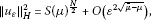(4.5)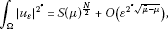(4.6)(4.7)

Lemma 4.4 

Suppose that () holds, $f\left(\tau \right)$ is defined as in (1.6) and ${V}_{\mu ,\epsilon }\left(x\right)$ are the minimizers of $S\left(\mu \right)$ defined as in (1.4). Then ${S}_{\eta ,\beta }\left(\mu \right)=f\left({\tau }_{0}\right)S\left(\mu \right)$ and has the minimizers $\left({V}_{\mu ,\epsilon }\left(x\right),{\tau }_{0}{V}_{\mu ,\epsilon }\left(x\right)\right)$, where $f\left({\tau }_{0}\right):={min}_{\tau \ge 0}f\left(\tau \right)>0$.

Lemma 4.5 Under the assumptions of Theorem 1.2, there exist $\stackrel{˜}{z}\in E\setminus \left\{0\right\}$ and ${\mathrm{\Lambda }}^{\ast }>0$ such that for all ${\sigma }_{1}+{\sigma }_{2}<{\mathrm{\Lambda }}^{\ast }$ there holds

$\underset{t\ge 0}{sup}J\left(t\stackrel{˜}{z}\right)<{c}^{\ast }=\frac{1}{N}{\left({S}_{\eta ,\beta }\left(\mu \right)\right)}^{\frac{N}{2}}+F\left({\tau }_{0}^{\left(1\right)},{\tau }_{0}^{\left(2\right)}\right).$
(4.8)

In particular, ${\alpha }_{\sigma }^{-}<{c}^{\ast }$ for all ${\sigma }_{1}+{\sigma }_{2}<{\mathrm{\Lambda }}^{\ast }$.

Proof For all $t\ge 0$, define the functions ${g}_{1}\left(t\right):=J\left(t{u}_{\epsilon },t{\tau }_{0}{u}_{\epsilon }\right)$ and

${g}_{2}\left(t\right):=\frac{{t}^{2}}{2}\left(1+{\left({\tau }_{0}\right)}^{2}\right){\parallel {u}_{\epsilon }\parallel }_{H}^{2}-\frac{{t}^{{2}^{\ast }}}{{2}^{\ast }}\left({\eta }_{1}{\left({\tau }_{0}\right)}^{{\beta }_{1}}+{\eta }_{2}{\left({\tau }_{0}\right)}^{{\beta }_{2}}\right){\int }_{\mathrm{\Omega }}{|{u}_{\epsilon }|}^{{2}^{\ast }}.$

Note that ${lim}_{t\to +\mathrm{\infty }}{g}_{2}\left(t\right)=-\mathrm{\infty }$ and ${g}_{2}\left(t\right)>0$ as t is closed to 0. Thus, ${sup}_{t\ge 0}{g}_{2}\left(t\right)$ is attained at some finite ${t}_{\epsilon }>0$ with ${g}_{2}^{\prime }\left({t}_{\epsilon }\right)=0$. Furthermore, ${C}^{\prime }<{t}_{\epsilon }<{C}^{″}$, where ${C}^{\prime }$ and ${C}^{″}$ are the positive constants independent of ε.

Choose ${\delta }_{1}>0$ small enough such that ${c}^{\ast }>0$ for all ${\sigma }_{1}+{\sigma }_{2}<{\delta }_{1}$. Set ${z}_{\epsilon }=\left({u}_{\epsilon },{\tau }_{0}{u}_{\epsilon }\right)$. Then $J\left(t{z}_{\epsilon }\right)\le \frac{{t}^{2}}{2}{\parallel {z}_{\epsilon }\parallel }^{2}$ for all $t\ge 0$ and ${\sigma }_{1},{\sigma }_{2}>0$, which implies that there exists ${t}_{0}\in \left(0,1\right)$ satisfying ${sup}_{0\le t\le {t}_{0}}J\left(t{z}_{\epsilon }\right)<{c}^{\ast }$, for all ${\sigma }_{1}+{\sigma }_{2}<{\delta }_{1}$. Note that

$\underset{t\ge 0}{max}\left(\frac{{t}^{2}}{2}{B}_{1}-\frac{{t}^{{2}^{\ast }}}{{2}^{\ast }}{B}_{2}\right)=\frac{1}{N}{\left({B}_{1}{B}_{2}^{-\frac{2}{{2}^{\ast }}}\right)}^{\frac{N}{2}},\phantom{\rule{1em}{0ex}}{B}_{1},{B}_{2}>0.$
(4.9)

From (4.9) and Lemmas 4.3, 4.4 it follows that

$\begin{array}{rl}{g}_{2}\left({t}_{\epsilon }\right)& \le \frac{1}{N}{\left(\frac{\left(1+{\left({\tau }_{0}\right)}^{2}\right){\parallel {u}_{\epsilon }\parallel }_{H}^{2}}{{\left(\left({\eta }_{1}{\left({\tau }_{0}\right)}^{{\beta }_{1}}+{\eta }_{2}{\left({\tau }_{0}\right)}^{{\beta }_{2}}\right){\int }_{\mathrm{\Omega }}{|{u}_{\epsilon }|}^{{2}^{\ast }}\right)}^{\frac{2}{{2}^{\ast }}}}\right)}^{\frac{N}{2}}\\ \le \frac{1}{N}{\left(f\left({\tau }_{0}\right)\frac{S{\left(\mu \right)}^{\frac{N}{2}}+O\left({\epsilon }^{2\sqrt{\overline{\mu }-\mu }}\right)}{{\left(S{\left(\mu \right)}^{\frac{N}{2}}+O\left({\epsilon }^{{2}^{\ast }\sqrt{\overline{\mu }-\mu }}\right)\right)}^{\frac{2}{{2}^{\ast }}}}\right)}^{\frac{N}{2}}\\ \le \frac{1}{N}{\left(f\left({\tau }_{0}\right)S\left(\mu \right)\right)}^{\frac{N}{2}}+O\left({\epsilon }^{2\sqrt{\overline{\mu }-\mu }}\right)\\ =\frac{1}{N}{\left({S}_{\eta ,\beta }\left(\mu \right)\right)}^{\frac{N}{2}}+O\left({\epsilon }^{2\sqrt{\overline{\mu }-\mu }}\right).\end{array}$

Consequently,

$\begin{array}{rcl}\underset{t\ge {t}_{0}}{sup}{g}_{1}\left(t\right)& <& \underset{t\ge {t}_{0}}{sup}\left({g}_{2}\left(t\right)-\frac{{t}^{q}}{q}K\left({z}_{\epsilon }\right)\right)\\ \le & \frac{1}{N}{\left({S}_{\eta ,\beta }\left(\mu \right)\right)}^{\frac{N}{2}}+O\left({\epsilon }^{2\sqrt{\overline{\mu }-\mu }}\right)-\frac{{t}_{0}^{q}}{q}\left({\sigma }_{1}+{\left({\tau }_{0}\right)}^{q}{\sigma }_{2}\right){\int }_{\mathrm{\Omega }}{|{u}_{\epsilon }|}^{q}\\ \le & \frac{1}{N}{\left({S}_{\eta ,\beta }\left(\mu \right)\right)}^{\frac{N}{2}}+O\left({\epsilon }^{2\sqrt{\overline{\mu }-\mu }}\right)-C\left({\sigma }_{1}+{\sigma }_{2}\right){\int }_{\mathrm{\Omega }}{|{u}_{\epsilon }|}^{q}\\ \le & \frac{1}{N}{\left({S}_{\eta ,\beta }\left(\mu \right)\right)}^{\frac{N}{2}}+O\left({\epsilon }^{2\sqrt{\overline{\mu }-\mu }}\right)-\left({\sigma }_{1}+{\sigma }_{2}\right){O}_{1}\left({\epsilon }^{N-q\sqrt{\overline{\mu }}}\right)\end{array}$

and

$\left(N-q\sqrt{\overline{\mu }}\right)\frac{2-q}{q}<2\sqrt{\overline{\mu }-\mu }-\left(N-q\sqrt{\overline{\mu }}\right),$

where we have used the assumption $\mu <\overline{\mu }-{\left(\frac{N}{q}-\sqrt{\overline{\mu }}\right)}^{2}$.

Therefore we can choose ${\sigma }_{1}={O}_{1}\left({\epsilon }^{{r}_{1}}\right)$, ${\sigma }_{2}={O}_{1}\left({\epsilon }^{{r}_{2}}\right)$ such that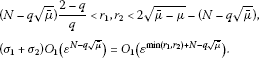The definition of $F\left({\tau }_{0}^{\left(1\right)},{\tau }_{0}^{\left(2\right)}\right)$ in Lemma 2.1 implies that

$F\left({\tau }_{0}^{\left(1\right)},{\tau }_{0}^{\left(2\right)}\right)=-{O}_{1}\left({\epsilon }^{\frac{2}{2-q}min\left({r}_{1},{r}_{2}\right)}\right).$

Note that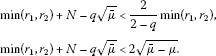Taking ε small enough, there exists ${\delta }_{2}>0$ such that for all $0<{\sigma }_{1}+{\sigma }_{2}<{\delta }_{2}$,

$O\left({\epsilon }^{2\sqrt{\overline{\mu }-\mu }}\right)-\left({\sigma }_{1}+{\sigma }_{2}\right){O}_{1}\left({\epsilon }^{N-q\sqrt{\overline{\mu }}}\right)
(4.10)

Choose ${\mathrm{\Lambda }}^{\ast }=min\left\{{\delta }_{1},{\delta }_{2}\right\}>0$. Then for all ${\sigma }_{1}+{\sigma }_{2}\in \left(0,{\mathrm{\Lambda }}^{\ast }\right)$ there holds

$\underset{t\ge 0}{sup}J\left(t{z}_{\epsilon }\right)<{c}^{\ast }.$
(4.11)

Finally, we prove that ${\alpha }_{\sigma }^{-}<{c}^{\ast }$ for all ${\sigma }_{1}+{\sigma }_{2}<{\mathrm{\Lambda }}^{\ast }$. Recall that ${z}_{\epsilon }=\left({u}_{\epsilon },{\tau }_{0}{u}_{\epsilon }\right)$. By Lemma 3.5, the definition of ${\alpha }_{\sigma }^{-}$ and (4.11), we can deduce that there exists ${\stackrel{˜}{t}}_{0}>0$ such that ${\stackrel{˜}{t}}_{0}{z}_{\epsilon }\in {\mathcal{M}}_{\sigma }^{-}$ and

${\alpha }_{\sigma }^{-}\le J\left({\stackrel{˜}{t}}_{0}{z}_{\epsilon }\right)\le \underset{t\ge 0}{sup}J\left({\stackrel{˜}{t}}_{0}{z}_{\epsilon }\right)<{c}^{\ast }.$

The proof is thus complete by taking $\stackrel{˜}{z}={z}_{\epsilon }$. □

Lemma 4.6 Set ${\mathrm{\Upsilon }}_{2}:=min\left\{{\mathrm{\Lambda }}^{\ast },\frac{q}{2}{\mathrm{\Upsilon }}_{1}\right\}$. Then for all ${\sigma }_{1}+{\sigma }_{2}\in \left(0,{\mathrm{\Upsilon }}_{2}\right)$, problem (1.1) has a positive solution ${z}^{\left(2\right)}$ such that ${z}^{\left(2\right)}\in {\mathcal{M}}_{\sigma }^{-}$ and $J\left({z}^{\left(2\right)}\right)={\alpha }_{\sigma }^{-}$.

Proof By Lemma 4.1, there exists a ${\text{(PS)}}_{{\alpha }_{\sigma }^{-}}$-sequence $\left\{{z}_{n}\right\}\subset {\mathcal{M}}_{\sigma }^{-}$ of J for all ${\sigma }_{1}+{\sigma }_{2}<\frac{q}{2}{\mathrm{\Upsilon }}_{1}$. From Lemmas 2.3, 3.4 and 4.5, it follows that ${\alpha }_{\sigma }^{-}>0$ and J satisfies the ${\text{(PS)}}_{{\alpha }_{\sigma }^{-}}$ condition for all ${\sigma }_{1}+{\sigma }_{2}<{\mathrm{\Upsilon }}_{2}$. Since J is coercive on ${\mathcal{M}}_{\sigma }$, we get that $\left\{{z}_{n}\right\}$ is bounded in E. Therefore, there exist a subsequence (still denoted by $\left\{{z}_{n}\right\}$) and ${z}^{\left(2\right)}=\left({u}^{\left(2\right)},{v}^{\left(2\right)}\right)\in {\mathcal{M}}_{\sigma }^{-}$ such that ${z}_{n}\to {z}^{\left(2\right)}$ strongly in E and $J\left({z}^{\left(2\right)}\right)={\alpha }_{\sigma }^{-}>0$ for all ${\sigma }_{1}+{\sigma }_{2}<{\mathrm{\Upsilon }}_{2}$. Since $J\left({z}^{\left(2\right)}\right)=J\left(|{z}^{\left(2\right)}|\right)$ and $|{z}^{\left(2\right)}|\in {\mathcal{M}}_{\sigma }^{-}$, by Lemma 3.2 we may assume that ${z}^{\left(2\right)}$ is a nontrivial nonnegative solution of (1.1). Moreover, by (3.7) and ${z}^{\left(2\right)}=\left({u}^{\left(2\right)},{v}^{\left(2\right)}\right)\in {\mathcal{M}}_{\sigma }^{-}$, we get

$Q\left({z}^{\left(2\right)}\right)=\frac{2-q}{{2}^{\ast }-q}{\parallel {z}^{\left(2\right)}\parallel }^{2}>0.$

This implies that ${u}^{\left(2\right)}\not\equiv 0$ and ${v}^{\left(2\right)}\not\equiv 0$. From the strong maximum principle  it follows that ${z}^{\left(2\right)}$ is a positive solution of (1.1). □

Proof of Theorems 1.1 and 1.2. By Lemma 4.2, we obtain that (1.1) has a positive solution ${z}^{\left(1\right)}\in {\mathcal{M}}_{\sigma }^{+}$ for all ${\sigma }_{1}+{\sigma }_{2}<{\mathrm{\Upsilon }}_{1}$. On the other hand, from Lemma 4.6, we can get the second positive solution ${z}^{\left(2\right)}\in {\mathcal{M}}_{\sigma }^{-}$ for all ${\sigma }_{1}+{\sigma }_{2}<{\mathrm{\Upsilon }}_{2}<{\mathrm{\Upsilon }}_{1}$. Since ${\mathcal{M}}_{\sigma }^{+}\cap {\mathcal{M}}_{\sigma }^{-}=\mathrm{\varnothing }$, this implies that ${z}^{\left(1\right)}$ and ${z}^{\left(2\right)}$ are distinct. □

## References

1. Rabinowitz P CBMS Regional Conference Series in Mathematics 65. In Minimax Methods in Critical Point Theory with Applications to Differential Equations. Am. Math. Soc, Providence; 1986.

2. Hardy G, Littlewood J, Polya G: Inequalities. Cambridge University Press, Cambridge; 1952.

3. Terracini S: On positive solutions to a class of equations with a singular coefficient and critical exponent. Adv. Differ. Equ. 1996, 2: 241-264.

4. Kang D, Peng S: Solutions for semilinear elliptic problems with critical Sobolev-Hardy exponents and Hardy potential. Appl. Math. Lett. 2005, 18: 1094-1100. 10.1016/j.aml.2004.09.016

5. Cao D, Kang D: Solutions of quasilinear elliptic problems involving a Sobolev exponent and multiple Hardy-type terms. J. Math. Anal. Appl. 2007, 333: 889-903. 10.1016/j.jmaa.2006.12.005

6. Kang D: On the quasilinear elliptic problems with critical Sobolev-Hardy exponents and Hardy terms. Nonlinear Anal. 2008, 68: 1973-1985. 10.1016/j.na.2007.01.024

7. Alves C, Filho D, Souto M: On systems of elliptic equations involving subcritical or critical Sobolev exponents. Nonlinear Anal. 2000, 42: 771-787. 10.1016/S0362-546X(99)00121-2

8. Abdellaoui B, Felli V, Peral I:Some remarks on systems of elliptic equations doubly critical in the whole ${\mathbb{R}}^{N}$. Calc. Var. Partial Differ. Equ. 2009, 34: 97-137. 10.1007/s00526-008-0177-2

9. Bouchekif M, Nasri Y: On elliptic system involving critical Sobolev-Hardy exponents. Mediterr. J. Math. 2008, 5: 237-252. 10.1007/s00009-008-0147-0

10. Bouchekif M, Nasri Y: On a singular elliptic system at resonance. Ann. Mat. Pura Appl. 2010, 189: 227-240. 10.1007/s10231-009-0106-9

11. Cai M, Kang D: Elliptic systems involving multiple strongly-coupled critical terms. Appl. Math. Lett. 2012, 25: 417-422. 10.1016/j.aml.2011.09.026

12. Hsu TS: Multiplicity of positive solutions for critical singular elliptic systems with concave-convex nonlinearities. Adv. Nonlinear Stud. 2009, 9: 295-311.

13. Huang Y, Kang D: On the singular elliptic systems involving multiple critical Sobolev exponents. Nonlinear Anal. 2011, 74: 400-412. 10.1016/j.na.2010.08.051

14. Huang Y, Kang D: Elliptic systems involving the critical exponents and potentials. Nonlinear Anal. 2009, 71: 3638-3653. 10.1016/j.na.2009.02.024

15. Liu Z, Han P: Existence of solutions for singular elliptic systems with critical exponents. Nonlinear Anal. 2008, 69: 2968-2983. 10.1016/j.na.2007.08.073

16. Wang L, Wei Q, Kang D: Existence and multiplicity of positive solutions to elliptic systems involving critical exponents. J. Math. Anal. Appl. 2011, 383: 541-552. 10.1016/j.jmaa.2011.05.053

17. Brézis H, Lieb E: A relation between pointwise convergence of functions and convergence of functionals. Proc. Am. Math. Soc. 1983, 88: 486-490.

18. Han P: The effect of the domain topology on the number of positive solutions of elliptic systems involving critical Sobolev exponents. Houst. J. Math. 2006, 32: 1241-1257.

19. Brown KJ, Zhang Y: The Nehari manifold for a semilinear elliptic equation with a sign-changing weigh function. J. Differ. Equ. 2003, 193: 481-499. 10.1016/S0022-0396(03)00121-9

20. Brown KJ, Wu TF: A semilinear elliptic system involving nonlinear boundary condition and sign-changing weigh function. J. Math. Anal. Appl. 2008, 337: 1326-1336. 10.1016/j.jmaa.2007.04.064

21. Wu TF: On semilinear elliptic equations involving concave-convex nonlinearities and sign-changing weight function. J. Math. Anal. Appl. 2006, 318: 253-270. 10.1016/j.jmaa.2005.05.057

22. Vazquez J: A strong maximum principle for some quasilinear elliptic equations. Appl. Math. Optim. 1984, 12: 191-202. 10.1007/BF01449041

## Author information

Authors

### Corresponding author

Correspondence to Tsing-San Hsu.

### Competing interests

The author declares that he has no competing interests.

## Rights and permissions

Reprints and Permissions

Hsu, TS. Existence and multiplicity of positive solutions to a perturbed singular elliptic system deriving from a strongly coupled critical potential. Bound Value Probl 2012, 116 (2012). https://doi.org/10.1186/1687-2770-2012-116

• Accepted:

• Published:

• DOI: https://doi.org/10.1186/1687-2770-2012-116

### Keywords

• Palais-Smale condition
• Nehari manifold
• strongly coupled
• elliptic system
• critical potential